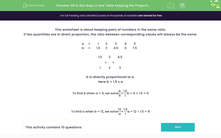# Find Missing Values Using Direct Proportion

In this worksheet, students will find the missing numbers in tables showing pairs in direct proportion.Key stage:  KS 3

Curriculum topic:   Ratio, Proportion and Rates of Change

Curriculum subtopic:   Solve Problems Involving Direct and Inverse Proportion

Popular topics:   Ratio worksheets

Difficulty level:#### Worksheet Overview

This activity is about keeping pairs of numbers in the same ratio.

If two quantities are in direct proportion, the ratio between corresponding values will always be the same.

 a = 1 2 3 4 5 b = 1.5 3 4.5 6 7.5

In this table, the ratio between a and b can be found by dividing the value of b by its corresponding value of a.

Where B is 3 and A is 2:   3 ÷ 2 = 1.5

Where B is 4.5 and A is 3:  4.5 ÷ 3 = 1.5

Now we know that the ratio between a and b is 1.5

If we know a and we want to find b, we simply multiply a by 1.5

For example, if a = 6 then b = 1.5 × 6 = 9

We can also use this ratio to find the value of a if we know what b is.

We do the inverse and simply divide b by 1.5

For example if b = 15, then a = 15 ÷ 1.5 = 10The best way to check that we've got this is to try some questions, so let's get started.

### What is EdPlace?

We're your National Curriculum aligned online education content provider helping each child succeed in English, maths and science from year 1 to GCSE. With an EdPlace account you’ll be able to track and measure progress, helping each child achieve their best. We build confidence and attainment by personalising each child’s learning at a level that suits them.

Get started